# Sampling and Sampling Distributions

## M1100, Section 3, November 11, 2002

This is a copy of the Mathematica notebook on which the November 11 lecture was based. Unfortunately, the web version is not interactive.

### Drawing a single sample from a population

##### Introduction

Imagine a large jar containing red and black beads.  The problem that we want to think about is the relationship between the proportion of red beads in the jar and the proportion of red beads in a sample.

Assessment Task 1: Although we are imagining a jar of beads, this is only an analogy for more meaningful situations that you might encounter (e.g., in the news).  List some examples and draw the analogy explicitly.  (For a hint, expand this cell.)

For example: someone wants to predict the outcome of an election by means of an exit poll.  All the people who voted are analogous to all the beads in the jar.  The color of bead is analogous to the vote---e.g. color:bead::candidate voted for:voter.  The people who are questioned in the poll are analogous to the sample.

Assessment Task 2: Suppose a large population is 40% red.  Imagine that you have drawn a sample of size 20 from this population.  Describe what you think a typical sample might be like.

Assessment Task 3: Suppose that you have drawn a sample of size 20 from a population of unknown proportion red, and that our sample is 40% red.  What do you think you cn deduce about the population?

##### The computer makes a population, and we examine a sample.

Now, we shall ask the computer to create a large population of individuals in which some fraction is red.  The computer will know that fraction, but we won't.

````We decide how large a sample we wish to take:

``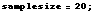``

And then we  ask the computer to draw a sample of that size:

````The computer will count up the proportion of red in the sample:

````
`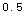`

Assessment Task 4: Now that you have seen a sample from the population in the computer's memory, what do think the proportion red is?

Assessmant Task 5: What do you think you know about the population?

##### We look at a few samples of various size.

Type the desired sample size in the input lines below, and then enter the cell.  The computer will create a sample, and will tell you the percent red in that sample.  Each time you enter the cell, a new sample is created and counted.

````
``

Assessment Task 6: Using the ability to draw samples of many sizes, determine the population proportion as best you can.   How accurate do you think your estimate is?

##### We  ask the computer to reveal the proportion red in the original population:
````
``

### Drawing many samples

##### Picking our own population and thinking about many samples.

This time, we will create a large population of individuals in which a fraction that we decide is red.  (Fill in the choice for the proportion below and enter the cell.)

````We are going to look at samples from this population.  Let us stipulate how large the samples will be:

````

We will ask the computer to pull out many samples of that size, and for each sample to show the proportion red in that sample.  We stipulate how many different samples we will ask the computer to make.

``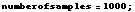``

Assessment Task 7: Instead of using a computer to do this, we could use a large bin with pieces of paper---or a jar filled with colored beads.  Describe what we would be doing if we were doing this task with physical manipulatives rather than on a computer.

Assessment Task 8: Before we ask the computer to draw all these samples, try to predict what the distribution of the sample statistic will look like.  How many samples will have a statistic of exactly p (the population proportion that you decided to work with)? What will the greatest deviation from p be?

By entering the next cell, we get the computer to create all the smples and reckon the statistic associated with each one.

``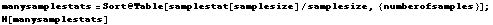``
`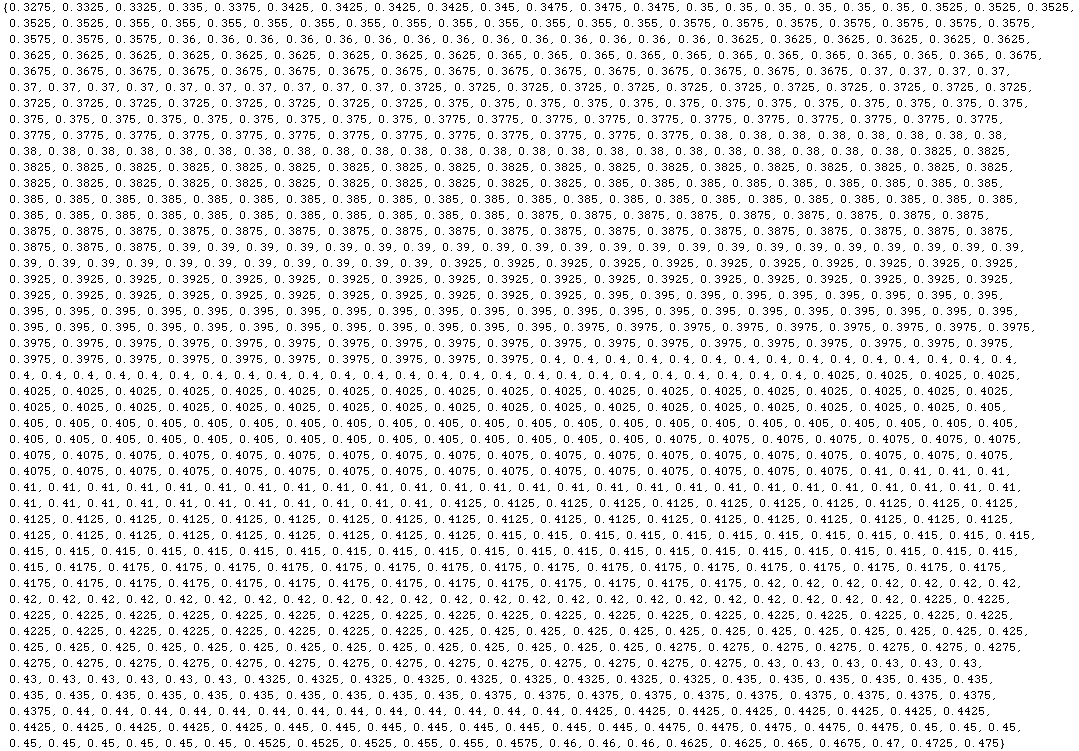`
##### The distribution of the statistic among all the samples we made.

We ask the comuter to tabulate this information we created in the last group:

````
 sample satistic 0.33 0.34 0.35 0.36 0.37 0.38 0.39 0.40 0.41 0.42 0.43 0.44 0.45 0.46 0.47 0.48
 # samples 1 4 14 41 57 97 138 162 161 136 89 49 35 9 5 2

The computer can also display it. The chart below shows the number of samples with various proportions of red.  (Proportion red is along the bottom.)

``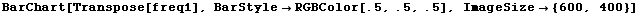``Assessment Task 9: Describe how the shape of the sampling distribution depends on the sample size.  (To do this, you will have to experiment with different sample sizes.)

Converted by Mathematica      November 12, 2002